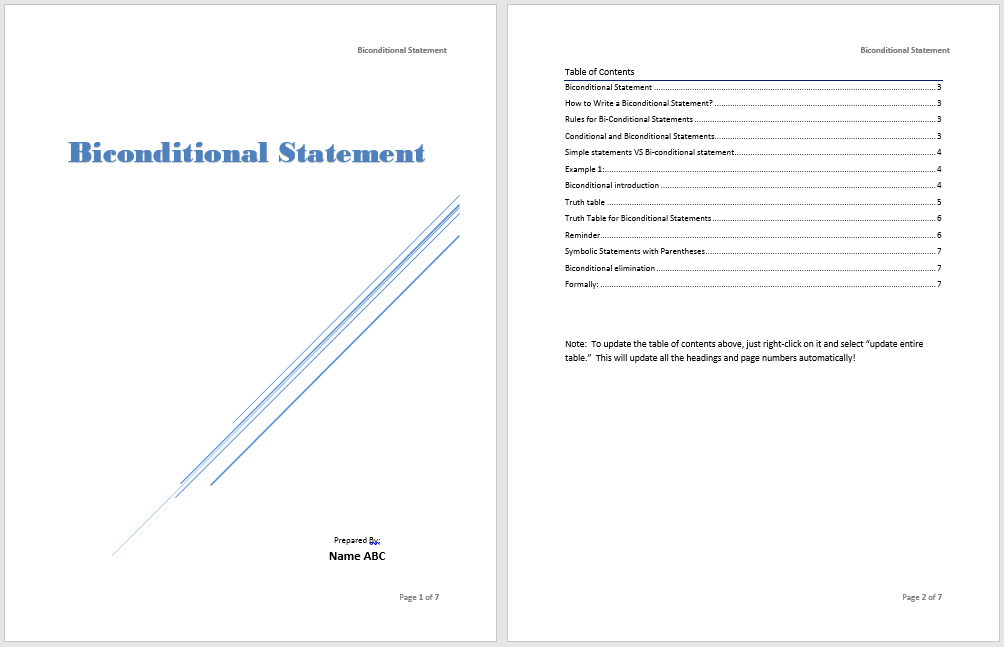# Bi-conditional Statement Template

A Bi-conditional statement is a mathematical and logical construct according to which, one statement is true if and only if another statement is true. These kinds of statements are used in the geometric and formal logic proofs. The truth and false of the statements is based on the truth and false of both statements. If the both statements are true, the table will be true, and if the statement is false, the table will also be false. De Morgan’s law is used to negate the bi-conditional statement that states the connection of logical operators is made by their negatives. The bi-conditional statement can be demonstrated through a common way by using its equivalence to the conjunction of the two converses. Both are demonstrated separately.

Rules of Inference

The biconditional has rules of inference just like all connectives in the first-order logic. It has governed uses as formal proofs:

Introduction of Bi-conditional

The Bi-conditional statement is inferred in a case that if Z follows from X, and X follows from Z, then X if and only if Z.

For example, from the statements “If I am eating, then I am hungry” and if I am hungry, then I am eating”, it can be inferred that “I am eating if and only if I am hungry” and “if I am hungry, then I am eating” or, equally inferable, “I am eating if and only if I am hungry”.

Z → XX → Z∴  X ↔ Z Z → XX → Z∴  Z ↔ X

Biconditional Elimination

Biconditional elimination makes it easy to infer the conditional from the biconditional: If (X ↔ Z), then the infer direction of the biconditional is (X → Z) and (Z → X). For instance, if it is true that “I am eating if and only if I am hungry” then it is also true that “if I am hungry, then I am eating”. Formally:

(X ↔ Z)∴ (X → Z)

Also

(X ↔ Z)∴ (Z → X)

Truth Tables for Bi-conditional

The truth or falsity statement is used as the representation statement in the truth tables. Truth table enables you to understand the combination and the truth and falsity of each statement. Truth table has a column for each statement to know about the false and truth of each compound statement:

• If the X is true and Z is true, then the both biconditional statements are true.
• If the X is true and Z is false, then the both biconditional statements are false.
• If X is false and Z is true, then the binconditional statements are false.
• If the X is false and Z is also false, the biconditional statements are true.

When a conditional statement and its converse are true, it can be written a bi-conditional statement. The conditional statement has one way arrow (→) and the bi-conditional statement has two way arrows (↔). If the both bi-conditional statements have same values, the compound statement will be true, otherwise it will be false.

Here is preview of a Free Sample Bi-conditional Statement Template created using MS Word,